Percentage Button - Techist - Tech Forum

 Register FAQ Members List Calendar Search Today's Posts Mark Forums Read Log in
 Thread Tools Display Modes11-13-2009, 04:16 AM   #1 (permalink)
-Deactivated-

Join Date: Dec 2006
Location: England
Posts: 5,723Percentage Button

I can not figure out how to do the percentage button, if someone could show me how to do it (using the same kind of code i've already used, not to complex) that would be greatIt's all done in VB6, and obviously there all actually seperated by whatever button each chunk of code works with. Sorry if it looks more confusing than it actually is !

Basically i have all the number buttons from 0 to 9, the +, - , divide, sqaure root, answer, clear and period button all working together (well the decimal button will only work to 1 decimal place) We have to recreate the Microsoft Windows Calculator for school work, so it's gotta work very similar to that.

Here is my current code.. it's kinda long.

Quote:
 Dim b As Integer Dim c As Integer Dim a As Integer Dim Number As Double Dim Number2 As Double Dim Number3 As Double Dim Number4 As Double Dim Number5 As Double Dim Number6 As Double Dim Number7 As Double Dim Number8 As Double Dim Number9 As Double Dim Number10 As Integer Dim Number11 As Integer Dim Number12 As Integer Dim Number13 As Integer Dim Number14 As Integer Dim Number15 As Integer Dim d As Integer 'Imports System.Math Dim e As Integer Dim f As Integer Dim g As Integer Private Sub ans_Click() If (a = 2) Then Number2 = Text1 Text1 = Number + Number2 ElseIf (a = 1) Then Number4 = Text1 Text1 = Number3 - Number4 ElseIf (a = 3) Then Number6 = Text1 Text1 = Number5 * Number6 ElseIf (a = 4) Then Number8 = Text1 Text1 = Number7 / Number8 ElseIf (e = 1) Then Number10 = Text1 Text1 = Math.Sqr(Number10) ElseIf (f = 1) Then Number11 = Text1 End If End Sub Private Sub Command1_Click() Number = 0 Number2 = 0 Number3 = 0 Number4 = 0 Number5 = 0 Number6 = 0 Number7 = 0 Number8 = 0 Text1 = "0." End Sub Private Sub Command2_Click() If Text1 = "0." Then Text1 = "." Else x = Len(Text1) temp = Left(Text1, x + 1) Text1 = temp + "." End If End Sub Private Sub divide_Click() a = 4 Number7 = Text1 Text1 = 0 End Sub Private Sub eight_Click() If Text1 = "0." Then Text1 = "8." ElseIf (d = 0) Then x = Len(Text1) temp = Left(Text1, x - 1) Text1 = temp + "8" + "." ElseIf (d = 1) Then x = Len(Text1) temp = Left(Text1, x - 1) Text1 = temp + "." + "8" d = 0 End If End Sub Private Sub five_Click() If Text1 = "0." Then Text1 = "5." ElseIf (d = 0) Then x = Len(Text1) temp = Left(Text1, x - 1) Text1 = temp + "5" + "." ElseIf (d = 1) Then x = Len(Text1) temp = Left(Text1, x - 1) Text1 = temp + "." + "5" d = 0 End If End Sub Private Sub four_Click() If Text1 = "0." Then Text1 = "4." ElseIf (d = 0) Then x = Len(Text1) temp = Left(Text1, x - 1) Text1 = temp + "4" + "." ElseIf (d = 1) Then x = Len(Text1) temp = Left(Text1, x - 1) Text1 = temp + "." + "4" d = 0 End If End Sub Private Sub minus_Click() a = 1 Number3 = Text1 Text1 = 0 End Sub Private Sub nine_Click() If Text1 = "0." Then Text1 = "9." ElseIf (d = 0) Then x = Len(Text1) temp = Left(Text1, x - 1) Text1 = temp + "9" + "." ElseIf (d = 1) Then x = Len(Text1) temp = Left(Text1, x - 1) Text1 = temp + "." + "9" d = 0 End If End Sub Private Sub one_Click() If Text1 = "0." Then Text1 = "1." ElseIf (d = 0) Then x = Len(Text1) temp = Left(Text1, x - 1) Text1 = temp + "1" + "." ElseIf (d = 1) Then x = Len(Text1) temp = Left(Text1, x - 1) Text1 = temp + "." + "1" d = 0 End If End Sub Private Sub perc_Click() Number12 = Text1 Text1 = "0." Number13 = Number12 End Sub Private Sub plus_Click() a = 2 Number = Text1 Text1 = 0 End Sub Private Sub point_Click() d = 1 End Sub Private Sub seven_Click() If Text1 = "0." Then Text1 = "7." ElseIf (d = 0) Then x = Len(Text1) temp = Left(Text1, x - 1) Text1 = temp + "7" + "." ElseIf (d = 1) Then x = Len(Text1) temp = Left(Text1, x - 1) Text1 = temp + "." + "7" d = 0 End If End Sub Private Sub six_Click() If Text1 = "0." Then Text1 = "6." ElseIf (d = 0) Then x = Len(Text1) temp = Left(Text1, x - 1) Text1 = temp + "6" + "." ElseIf (d = 1) Then x = Len(Text1) temp = Left(Text1, x - 1) Text1 = temp + "." + "6" d = 0 End If End Sub Private Sub sqrt_Click() e = 1 End Sub Private Sub three_Click() If Text1 = "0." Then Text1 = "3." ElseIf (d = 0) Then x = Len(Text1) temp = Left(Text1, x - 1) Text1 = temp + "3" + "." ElseIf (d = 1) Then x = Len(Text1) temp = Left(Text1, x - 1) Text1 = temp + "." + "3" d = 0 End If End Sub Private Sub times_Click() a = 3 Number5 = Text1 Text1 = 0 End Sub Private Sub two_Click() If Text1 = "0." Then Text1 = "2." ElseIf (d = 0) Then x = Len(Text1) temp = Left(Text1, x - 1) Text1 = temp + "2" + "." ElseIf (d = 1) Then x = Len(Text1) temp = Left(Text1, x - 1) Text1 = temp + "." + "2" d = 0 End If End Sub Private Sub zero_Click() If Text1 = "0." Then Text1 = "0." ElseIf (d = 0) Then x = Len(Text1) temp = Left(Text1, x - 1) Text1 = temp + "0" + "." ElseIf (d = 1) Then x = Len(Text1) temp = Left(Text1, x - 1) Text1 = temp + "0" + "." d = 0 End If End Sub
__________________

__________________11-13-2009, 09:34 AM #2 (permalink) Seg Fault'nJoin Date: Jul 2005 Location: The dried husk where America came from Posts: 5,801Re: Percentage Button I don't think the window calculator has a percentage button... anyway... answer = (firstNumber * secondNumber) /100; __________________ __________________There are in order of increasing severity: lies, darn lies, statistics, and computer benchmarks. - diskinfo man page "Get your money and let's go to court!" - Craig Mudie, MS11-13-2009, 04:25 PM #3 (permalink) -Deactivated-   Join Date: Dec 2006 Location: England Posts: 5,723Re: Percentage Button Yes it does. I get the maths behind it, that is not the problem at all. What should happen for example is, say i enter the number 100. It appears in the textbox, i need a temp store to store this number, but when i press + (just like the windows calc) the text box goes to '0.', and thus my temp store of 100 is erased and replaced with 0 - which is my problem, what should happen is after the +, i enter another number and then press the percentage button. Whatever number i entered prior to the percentage button should take that percentage of the temp stored number, and add it on and the result should be displayed when = is pressed. __________________Thread ToolsShow Printable VersionEmail this Page Display ModesLinear ModeSwitch to Hybrid ModeSwitch to Threaded ModePosting Rules You may not post new threads You may not post replies You may not post attachments You may not edit your posts BB code is On Smilies are On [IMG] code is On HTML code is OffTrackbacks are On Pingbacks are On Refbacks are Off Forum RulesSimilar Threads Thread Thread Starter Forum Replies Last Post zillah Microsoft Windows and Software 0 07-10-2008 05:21 PM RoRol Overclocking and Modding 6 05-27-2008 10:22 AM xXxexpertxXx Overclocking and Modding 0 02-21-2008 09:04 PM» Recent Threads

•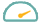Fuelly
•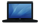Techist
•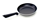Cooking Forum
•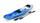Kayaking & Rafting Forum
•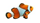Aquarium Forum
•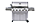BBQ Forum
•Early Retirement
•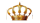Royal Forums
•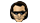U2 Music Forum
•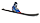Ski Forum
•Computer Forums
•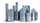CityProfile Local Forum

#### Our Communities

Our communities encompass many different hobbies and interests, but each one is built on friendly, intelligent membership.

» More about our Communities

•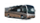iRV2 RV Forum
•Airstream Trailer Forum
•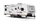Forest River Forums
•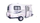Fiberglass RV Forums
•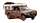Wander The West
•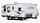Jayco RV Forum
•Luxury Coach Forum
•Escape Trailer Forum
•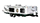Hilo Trailer Forum
•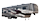5th Wheel Forums
•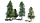RV Park Reviews
•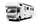Truck Conversions
•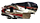Redwood Owners
•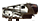Crossroads Owners
•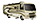Thor RV Forum
•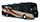Winnebago RV Forum
•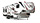Dutchmen RV Forum
•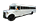School Bus Conversions
•Sunline Trailers Forum
•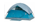Campground Reviews
•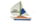Cruisers Sailing Forum
•Rigid Inflatable Boats
•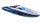Performance Boat Forum
•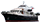Trawler Boating Forums
•Maxum Owners Club
•Sail Blogs
•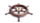Sailing Wiki
•Cruisers Log
•Yacht Blogs

#### Marine Communities

Our Marine websites focus on Cruising and Sailing Vessels, including forums and the largest cruising Wiki project on the web today.

» More about our Marine Communities